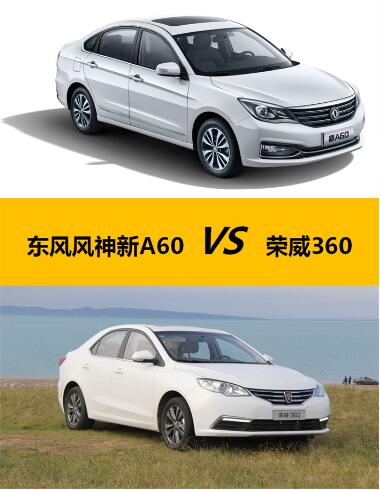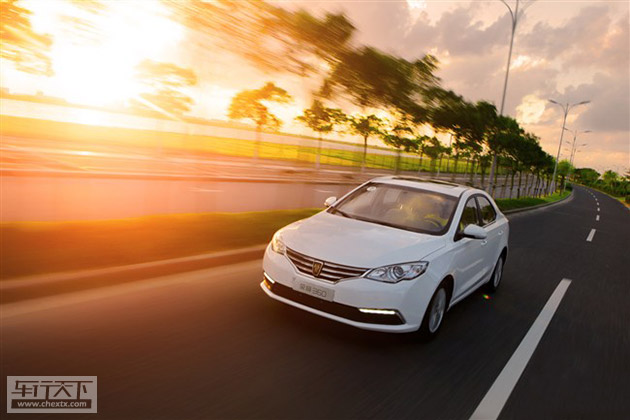## 大空间低油耗 两款超值自主家轿推荐

2016年03月22日 10:56 来源：www.chextx.com 超过：次关注

2016年的春节在一场倒春寒中正完全消失于记忆里，重返职场，熟悉的生活压力、工作压力扑面而来。开春的车市也充满了压力与竞争，但不少车企的产品都收获了开门红的局面。比如东风风神和上汽荣威的两款家轿，新A60和360两款车自上世以来，在销量上都有不错的表现，于今年1月共同跻身月销5000的A级车俱乐部。天气逐渐转暖，如果你想选一款好而不贵的车去踏春，不妨考虑下东风风神新A60和上汽荣威360。大空间一直都是车主们孜孜不倦的追求。东风风神新A60与上汽荣威360显然深谙这一点。两款车在空间方面表现都不错，新A60更胜一筹。

相较荣威360，东风风神新A60的超大车身更令人为之侧目，后排腿部空间达680mm，几乎达到了B级车的标准，极大提升了乘坐者的舒适度。后备箱空间表现上，东风风神新A60的后备箱容积达506L，比荣威360略大。前排的储物空间表现上，东风风神新A60中控下方小的储物格较多，非常方便驾驶员放些零散的东西。前排门板、手套箱、中央扶手箱等储物空间设计得较大较深，能放不少杂物。荣威360同样拥有这些储物空间，只是容积不如东风风神新A60设计的大。

东风风神新A60和荣威360起步价仅为7万元，但是配置都非常丰富。这也是为什么两款车一上市就引起大量关注的一大原因。

东风风神新A60搭载有丰富智能科技配置(MP5+智能互联+倒车影像)，安全配置方面，根据C-NCAP五星安全打造，整合ABS+EBD+BA和ESP等配置，轻松化解各种复杂路况，带来更安全的驾乘体验。并且，除了1.5L手动豪华型之外，其他车型都配有无钥匙进入与启动系统，逼格直升!荣威360号称要打造“360度全方位高品质”，配备inkaNet 4G智能网络行车系统、Loetsch第二代全息数字仪表盘、12键多功能方向盘以及8英寸触控屏，带给车主新颖的科技体验。安全性配置方面，荣威360标配了倒车雷达、BA刹车辅助等。并且全系标配胎压监测装置，也是十足的诚意!居家过日子，要面子也要里子。在油耗方面，东风风神新A60与荣威360“各显神通”，将百公里综合工况油耗都控制在了6L以内，为用户带来低成本长旅程的幸福节能新旅程。东风风神新A60则采用全新升级的E-TECO DFMA发动机和STT智能启停系统，再加上196N.m的最大输出扭矩，让新A60在综合工况下百公里油耗大大降低，节省了车主的日常用车成本。而荣威360作为上汽“蓝芯”战略的首款车型，搭载的“netblue蓝芯”动力总成，配以EPS电动助力转向系统、经济驾驶模式提示以及制动能量回收系统等燃油经济设计，实现百公里低油耗的经济驾驶。两款车1公里不到3毛钱的燃油经济性让人眼前一亮。

作为当前自主品牌A级车市场里的两款火爆车型，东风风神新A60和荣威360实力不相上下。如若家庭已成为您甜蜜的负担，那么拥有超长车身、更宽适空间的东风风神新A60想必更能让您和家人的休憩更随心。

#### 相关文章

﻿
• 快速找车
• 选择品牌
• 选择品牌
• A  奥迪
• A  阿斯顿·马丁
• A  阿尔法·罗密欧
• B  宝沃
• B  布加迪
• B  巴博斯
• B  保时捷
• B  宾利
• B  奔驰
• B  宝马
• B  本田
• B  别克
• B  标致
• B  比亚迪
• B  宝骏
• B  北汽制造
• B  北汽新能源
• B  北汽幻速
• B  北汽威旺
• B  北京汽车
• B  奔腾
• B  北汽绅宝
• B  北汽昌河
• C  长安欧尚
• C  长安
• C  长安凯程
• C  长城
• D  大众
• D  道奇
• D  DS
• D  东南
• D  东风风神
• D  东风风行
• D  东风小康
• D  东风风度
• D  东风
• F  福特
• F  丰田
• F  菲亚特
• F  法拉利
• F  福田
• F  福迪
• F  福汽启腾
• G  观致
• G  广汽传祺
• G  广汽吉奥
• G  GMC
• H  红旗
• H  汉腾汽车
• H  哈弗
• H  哈飞
• H  海格
• H  海马
• H  华颂
• H  黄海
• H  华泰
• H  恒天
• J  捷途
• J  几何汽车
• J  捷达
• J  吉利汽车
• J  捷豹
• J  Jeep
• J  江淮
• J  江铃
• J  金杯
• J  九龙
• J  金旅
• K  凯翼
• K  凯迪拉克
• K  克莱斯勒
• K  科尼塞克
• K  卡威
• K  开瑞
• L  路虎
• L  林肯
• L  劳斯莱斯
• L  兰博基尼
• L  雷克萨斯
• L  铃木
• L  领克
• L  雷诺
• L  理念
• L  力帆
• L  莲花汽车
• L  猎豹
• L  路特斯
• L  陆风
• M  马自达
• M  MG
• M  MINI
• M  玛莎拉蒂
• M  摩根
• M  迈凯轮
• N  纳智捷
• O  欧拉
• O  欧宝
• O  讴歌
• O  欧朗
• Q  奇瑞
• Q  起亚
• Q  启辰
• R  日产
• R  荣威
• R  瑞麒
• S  SERES赛力斯
• S  三菱
• S  斯威汽车
• S  萨博
• S  smart
• S  斯柯达
• S  斯巴鲁
• S  思铭
• S  双龙
• S  上汽大通
• S  双环
• T  特斯拉
• T  腾势
• W  蔚来
• W  沃尔沃
• W  WEY
• W  五菱汽车
• W  五十铃
• W  威兹曼
• W  威麟
• X  现代
• X  雪佛兰
• X  星途
• X  雪铁龙
• X  小鹏汽车
• X  西雅特
• Y  一汽
• Y  英菲尼迪
• Y  英致
• Y  依维柯
• Y  野马汽车
• Y  永源
• Z  众泰
• Z  中华
• Z  中兴
• Z  知豆
• 选择车系
• 选择车系
• 车型对比
• 选择品牌
• 选择品牌
• A  奥迪
• A  阿斯顿·马丁
• A  阿尔法·罗密欧
• B  宝沃
• B  布加迪
• B  巴博斯
• B  保时捷
• B  宾利
• B  奔驰
• B  宝马
• B  本田
• B  别克
• B  标致
• B  比亚迪
• B  宝骏
• B  北汽制造
• B  北汽新能源
• B  北汽幻速
• B  北汽威旺
• B  北京汽车
• B  奔腾
• B  北汽绅宝
• B  北汽昌河
• C  长安欧尚
• C  长安
• C  长安凯程
• C  长城
• D  大众
• D  道奇
• D  DS
• D  东南
• D  东风风神
• D  东风风行
• D  东风小康
• D  东风风度
• D  东风
• F  福特
• F  丰田
• F  菲亚特
• F  法拉利
• F  福田
• F  福迪
• F  福汽启腾
• G  观致
• G  广汽传祺
• G  广汽吉奥
• G  GMC
• H  红旗
• H  汉腾汽车
• H  哈弗
• H  哈飞
• H  海格
• H  海马
• H  华颂
• H  黄海
• H  华泰
• H  恒天
• J  捷途
• J  几何汽车
• J  捷达
• J  吉利汽车
• J  捷豹
• J  Jeep
• J  江淮
• J  江铃
• J  金杯
• J  九龙
• J  金旅
• K  凯翼
• K  凯迪拉克
• K  克莱斯勒
• K  科尼塞克
• K  卡威
• K  开瑞
• L  路虎
• L  林肯
• L  劳斯莱斯
• L  兰博基尼
• L  雷克萨斯
• L  铃木
• L  领克
• L  雷诺
• L  理念
• L  力帆
• L  莲花汽车
• L  猎豹
• L  路特斯
• L  陆风
• M  马自达
• M  MG
• M  MINI
• M  玛莎拉蒂
• M  摩根
• M  迈凯轮
• N  纳智捷
• O  欧拉
• O  欧宝
• O  讴歌
• O  欧朗
• Q  奇瑞
• Q  起亚
• Q  启辰
• R  日产
• R  荣威
• R  瑞麒
• S  SERES赛力斯
• S  三菱
• S  斯威汽车
• S  萨博
• S  smart
• S  斯柯达
• S  斯巴鲁
• S  思铭
• S  双龙
• S  上汽大通
• S  双环
• T  特斯拉
• T  腾势
• W  蔚来
• W  沃尔沃
• W  WEY
• W  五菱汽车
• W  五十铃
• W  威兹曼
• W  威麟
• X  现代
• X  雪佛兰
• X  星途
• X  雪铁龙
• X  小鹏汽车
• X  西雅特
• Y  一汽
• Y  英菲尼迪
• Y  英致
• Y  依维柯
• Y  野马汽车
• Y  永源
• Z  众泰
• Z  中华
• Z  中兴
• Z  知豆
• 选择车系
• 选择车系
• 选择车型
• 选择车型
• 意见反馈